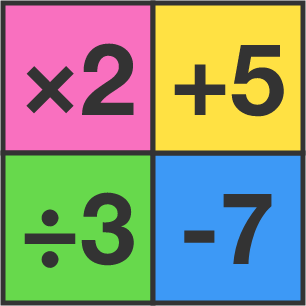# 57 of 100: Curious Calculator

Algebra Level 1You have a calculator with 4 buttons as shown; they multiply the current value shown on the calculator by 2, divide the current value by 3, add 5 to the current value, or subtract 7 from the current value.

If the screen starts at 6, what is the fewest button presses you need to make to get a value of 1?Hint: If working forwards from 6 is daunting, try working backwards from 1.

×